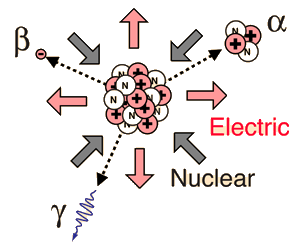Gamma radioactivity is composed of electromagnetic rays. It is distinguished from x-rays only by the fact that it comes from the nucleus. Most gamma rays are somewhat higher in energy than x-rays and therefore are very penetrating.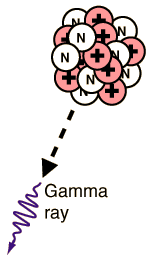Gamma radiation is the most useful type of radiation for medical purposes, but at the same time it is the most dangerous because of its ability to penetrate large thicknesses of material.

Index

 HyperPhysics***** Nuclear R Nave
Go Back

While the most common types of radioactive decay are by alpha, beta, and gamma radiation, several other varieties of radioactivity occur:

Electron capture: A parent nucleus may capture one of its own electrons and emit a neutrino. This is exhibited in the potassium-argon decay.

Positron or positive beta decay: Positron emission is called beta decay because the characteristics of electron or positron decay are similar. They both show a characteristic energy spectrum because of the emission of a neutrino or antineutrino.

Internal conversion is the use of electromagnetic energy from the nucleus to expel an orbital electron from the atom.

Index

 HyperPhysics***** Nuclear R Nave
Go Back

# Electron Capture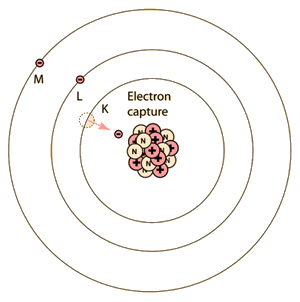Electron capture is one form of radioactivity. A parent nucleus may capture one of its orbital electrons and emit a neutrino. This is a process which competes with positron emission and has the same effect on the atomic number. Most commonly, it is a K-shell electron which is captured, and this is referred to as K-capture. This is a schematic that grossly distorts the picture relative to a scale model of the atom. The electron orbit radii are tens of thousands of times the diameter of the nucleus.
A typical example is the decay of beryllium

74Be + 0-1e73Li + ν

The capture of the electron by a proton in the nucleus is accompanied by the emission of a neutrino. The process leaves a vacancy in the electron energy level from which the electron came, and that vacancy is either filled by the dropping down of a higher-level electron with the emission of an X-ray or by the ejection of an outer electron in a process called the Auger effect.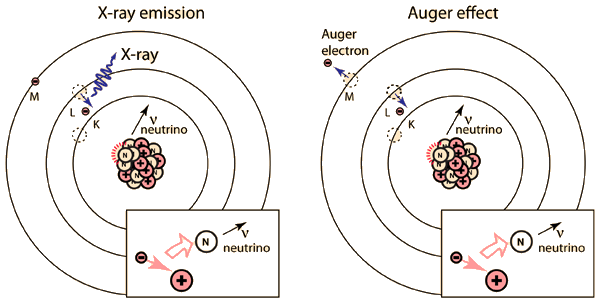Electron capture is a process which involves the weak interaction and can be represented by a Feynman diagram.

In the middle range of the periodic table, those isotopes which are lighter than the most stable isotopes tend to decay by electron capture, and those heavier decay by negative beta decay. An example of this pattern is seen with silver isotopes, with two stable isotopes plus one of lower mass which decays by electron capture and one of heavier mass which decays by beta emission.

An important example of electron capture decay is that of potassium-40 since it forms the basis for the potassium-argon mineral dating process.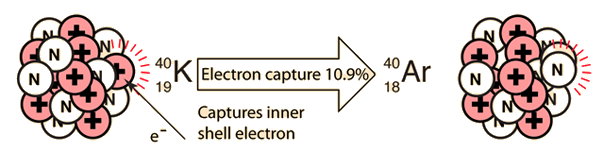Cobalt-57
Index

 HyperPhysics***** Nuclear R Nave
Go Back

# Internal Conversion

Internal conversion is another electromagnetic process which can occur in the nucleus and which competes with gamma emission. Sometimes the multipole electric fields of the nucleus interact with orbital electrons with enough energy to eject them from the atom. This process is not the same as emitting a gamma ray which knocks an electron out of the atom. It is also not the same as beta decay, since the emitted electron was previously one of the orbital electrons, whereas the electron in beta decay is produced by the decay of a neutron.An example used by Krane is that of 203Hg, which decays to 203Tl by beta emission, leaving the 203Tl in an electromagnetically excited state. It can proceed to the ground state by emitting a 279.190 keV gamma ray, or by internal conversion. In this case the internal conversion is more probable. Since the internal conversion process can interact with any of the orbital electrons, the result is a spectrum of internal conversion electrons which will be seen as superimposed upon the electron energy spectrum of the beta emission. The energy yield of this electromagnetic transition can be taken as 279.190 keV, so the ejected electrons will have that energy minus their binding energy in the 203Tl daughter atom.

The diagram above is of course conceptual only and not to scale since the nuclear radius of thallium is modeled to be about 0.7x10-14 m and the radius of the atom is about 1.76x10-10m, a factor of about 25,000 larger! And of course the planetary type orbits of the electrons are unrealistic since the wave properties of the electrons lead to charge distributions that give a finite probability that the K electron shown above will actually extend inside the nucleus so that the nucleus can interact with it and hand off its excess energy. An examination of the electron distribution for the simplest atom, hydrogen, can give the perspective that the electron has a small but finite probability of extending into the nucleus. From the table of binding energies below, you can see that the binding energy of the K-shell electron is over 85,000 electron volts compared to 13.6 eV for the hydrogen electron, or over 6,000 times larger.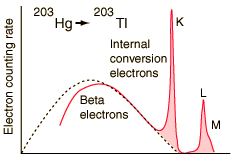Electron emissions from the Hg-203 to Tl-203 decay, measured by A. H. Wapstra, et al., Physica 20, 169 (1954).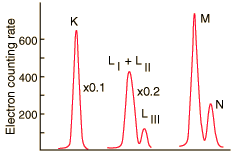At higher resolution, the internal conversion electrons from the L, M and N shells can be resolved. Z. Sujkowski, Ark. Fys. 20, 243 (1961).At even higher resolution, the three L shells can be resolved. From C. J. Herrlander and R. L. Graham, Nucl. Phys. 58, 544 (1964).

The resolution of the electron detection is good enough that such internal conversion electron spectra can be used to study the binding energies of the electrons in heavy atoms. In this case, the measured electron energies can be subtracted from the transition energy as indicated by the gamma emission, 279.190 keV.

 Binding energiesfor 203Tl K 85.529 keV LI 15.347 keV LII 14.698 keV LIII 12.657 keV M 3.704 keV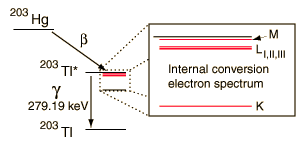In addition to information from the internal conversion electrons about the binding energies of the electrons in the daughter atom, the relative intensities of these internal conversion electron peaks can give information about the electric multipole character of the nucleus.

Index

Reference
Krane
Nuclear Physics, Sec 10.6

Internal conversion Wiki

 HyperPhysics***** Nuclear R Nave
Go Back